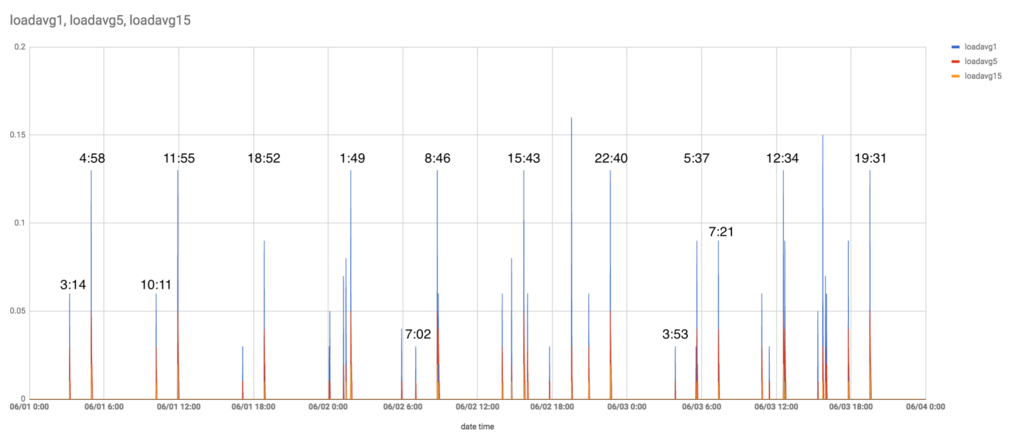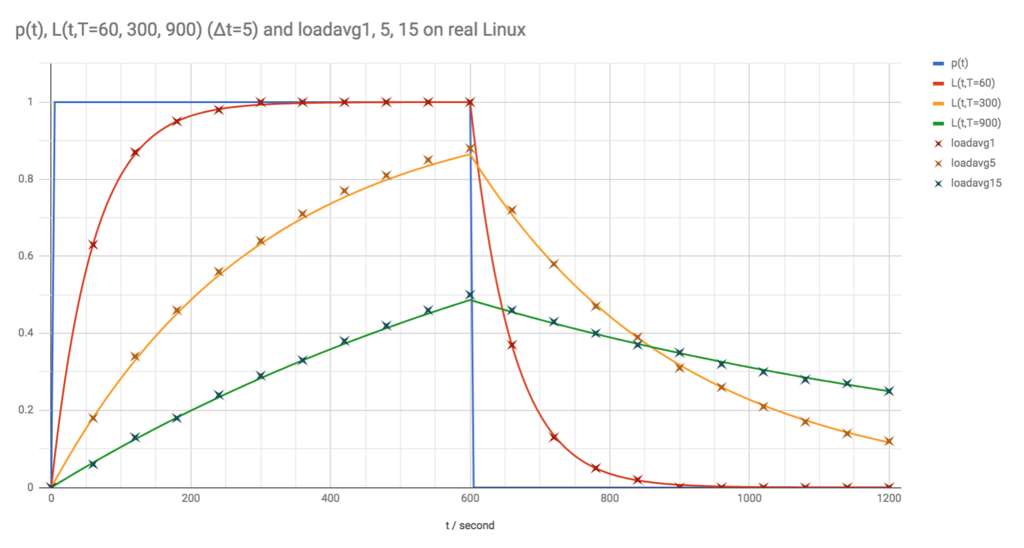# Understanding why the Linux loadavg rises every 7 hours

Mackerel team engineerid:itchyny here (Mackerel is a server monitoring service and mackerel-agent is a daemon program to collect and post server's metrics).

”When mackerel-agent is installed, the loadavg rises every 7 hours”

Recently, we’ve received several inquiries like the one above from multiple customers. So I tried it out for myself, and sure enough, this issue was reproduced. I installed mackerel-agent on EC2t2.micro, configured basic log and process monitoring, and left it for a few days.Indeed, the loadavg rose approximately every 7 hours. I did not configure cron for this cycle and no processing was done even within mackerel-agent. However, the loadavg's peak value increased the more plugins were added.

This entry explains the cause of this phenomenon.

To understand the reasons why the loadavg rises, we first need to understand how the loadavg itself is calculated. So let’s first go over how Linux calculates loadavg.

The Linux loadavg is an exponential moving average value of the total number of “runnable” processes in the run queue and disk I/O waited (uninterruptible) processes. The following are statuses of the Linux process.

 % man ps | grep -A 10 "^PROCESS STATE"
PROCESS STATE CODES
Here are the different values that the s, stat and state output specifiers (header "STAT" or "S") will display to describe the state of a process:

D    uninterruptible sleep (usually IO)
R    running or runnable (on run queue)
S    interruptible sleep (waiting for an event to complete)
T    stopped, either by a job control signal or because it is being traced
W    paging (not valid since the 2.6.xx kernel)
X    dead (should never be seen)
Z    defunct ("zombie") process, terminated but not reaped by its parent

The loadavg is the value that represents the number of processes with the statuses R and D smoothed by the exponential weighted average.

Let's express the total number of running and uninterruptible processes subject to the loadavg aswithas a function for time. If the sampling interval isand the time constant is, the exponential moving average of the number of processes, that is, the exponentially decaying weighted average can be written as follows 1.The exponential moving average value ofaccumulated over time can be expressed with the weighted average of the previously calculated valueand the current value. Even if you haven’t kept a history of the number of processes up until this point, we can calculate a new loadavg from the previous loadavg and the current number of processes.

Based on this recurrence formula, by selecting an appropriate constant and comparing the simulated value () and the actual value from Linux (Run yes > /dev/null& on EC2 t2.micro and drop that process after 10 minutes), the following results were obtained.Let’s confirm that the loadavg is actually being calculated based on the above recurrence formula while referring to the Linux source code. First, let’s look into kernel/sched/loadavg.c.

/* Variables and functions for calc_load */
unsigned long avenrun;

{
unsigned long sample_window;
long active, delta;

if (time_before(jiffies, sample_window + 10))
return;

// ...

active = active > 0 ? active * FIXED_1 : 0;

// ...
}


The loadavg values are stored in avenrun. We can see that in order for this value to periodically update, the value of calc_load_update is compared against jiffies (a variable representing time, increasing by 1 every tick) while increasing by LOAD_FREQ. In other words, loadavg is updated every LOAD_FREQ. As described in include/linux/sched/loadavg.h, this value is 5*HZ+1. Since HZ is the value of how many ticks increase per second 2, we can see that the loadavg is updated every 5 seconds plus one tick. Now let’s look at calc_load which is the actual value calculation.

/*
* a1 = a0 * e + a * (1 - e)
*/
static unsigned long
{

}


Ignore the branching statement and write it in one expression 3.

 avenrun = ((FIXED_1 - EXP_1) * active + EXP_1 * avenrun) / FIXED_1;


Here, according to include/linux/sched/loadavg.h, EXP_1 = 1884 and FIXED_1 = (1<<11). By substitutingfor the recurrence formula obtained above, we get following.Taking it into account that Linux uses values shifted by 11 bits (i.e. scaled by FIXED_1) to calculate only by integral operations, andequals to roughly 1884, we can see that the calculation in Linux indeed matches the recurrence formula. Loadavg 5, 15 can be checked in the same way.

The loadavg is updated approximately every 5 seconds using the number of processes in the run queue at that moment (calc_load_tasks) and the recurrence formula. To be exact, it is calculated every 5*HZ+1 ticks. This +1 is the cause for the loadavg rising roughly every 7 hours.

mackerel-agent opens the plugin processes to collect metrics every minute. Since recalculation of the loadavg takes place approximately every 5 seconds, the timing of when mackerel-agent starts collecting metrics and the timing of the loadavg recalculation will periodically be in sync.

In an environment where HZ is 1000, the loadavg is updated every 5.001 seconds. When multiplied by 5000, the cycle is 25005 seconds (or 6 hours, 56 minutes and 45 seconds) and the +1 accumulates and coincides with 5*HZ. This cycle is not a multiple of 60 seconds which is the metric collection interval of mackerel-agent, however, a phenomenon occurs when the timing of opening processes and the loadavg update roughly overlap (off by 0.003 seconds) every 6 hours and 57 minutes.

In an environment where HZ is 250, repeating 5*HZ+1 1250 times comes out to 6255 seconds (or 1 hour 44 minutes 15 seconds). It’s normal for the loadavg to go up during this cycle, but we can’t ignore the fact that the metric collection timing for mackerel-agent shifts by 0.012 seconds each cycle. The value is multiplied by 4, that is, 6 hours and 57 minutes, which is a multiple of 60 seconds and this overlaps perfectly with mackerel-agent 's metrics collection timing. In my own experience HZ equaled 250. If you look at the graph at the beginning of this entry, you can see that there are peaks of uniform height with a cycle of 6 hours 57 minutes. You can also see that there are smaller peaks 1 hour 44 minutes before and after these peaks. This is a phenomenon derived from HZ being 250.

So why isn’t the loadavg update interval exact 5 seconds? Well, in Linux, various processes are running in their own cycle. And if a process with a 5 second interval exists, there is a possibility that it may sync with the loadavg recalculation timing. In order to prevent this phenomenon of the loadavg unintentionally rising due to this timing coincidence, the cycle is slightly shifted from 5 seconds. For more details on this, take a look at the mailing list or the patch commit message where 1 was added to 5*HZ.

The loadavg update interval in Linux is intentionally slightly deviated from 5 seconds. This slight deviation accumulates and is a multiple of 60 seconds in a cycle of about 6 hours 57 minutes. mackerel-agent opens plugin processes every 60 seconds, and the timing of this periodically coincides with the loadavg recalculation. This is the cause of the phenomenon in which the loadavg periodically spikes when mackerel-agent is installed. This phenomenon has also been reported with other monitoring tools such as collectd and Telegraf

## References

1. You can verify that the sum of the coefficients is 1. This is a necessary condition for being a weighted average.

2. In this entry HZ refers to the kernel timer frequency CONFIG_HZ, which must be distinguished from the userland frequency USER_HZ. USER_HZ is the value obtained from getconf CLK_TCK and /proc/stat, which is defined as 100 on x86. The kernel timer frequency can be checked with grep "CONFIG_HZ=" /boot/config-\$(uname -r) or by watching the increment of watch -n1 "grep ^jiffies /proc/timer_list | head -n1".

3. The branching procedure is required so that a system with no processes has a loadavg of 0.0 and a system with one process has 1.0. If this procedure does not exist, the loadavg will never reach 1.0, and if added without branching, it will not reach 0.0 on idle. It used to be that the value was rounded off, but the problem of not reaching 0.0 in an idle state was identified and it now the value is rounded up when the number of processes increases. For details, refer to the patch and the mailing list.